# Exploratory Data Analysis

## Preface

After you have acquired the data, you should do the following:

• Diagnose data quality.
• If there is a problem with data quality,
• The data must be corrected or re-acquired.
• Explore data to understand the data and find scenarios for performing the analysis.
• Derive new variables or perform variable transformations.

The dlookr package makes these steps fast and easy:

• Performs an data diagnosis or automatically generates a data diagnosis report.
• Discover data in a variety of ways, and automatically generate EDA(exploratory data analysis) report.
• Imputate missing values and outliers, resolve skewed data, and binarize continuous variables into categorical variables. And generates an automated report to support it.

This document introduces EDA(Exploratory Data Analysis) methods provided by the dlookr package. You will learn how to EDA of `tbl_df` data that inherits from data.frame and `data.frame` with functions provided by dlookr.

dlookr synergy with `dplyr` increases. Particularly in data exploration and data wrangle, it increases the efficiency of the `tidyverse` package group.

## Supported data structures

Data diagnosis supports the following data structures.

• data frame : data.frame class.
• data table : tbl_df class.
• table of DBMS : table of the DBMS through tbl_dbi.
• Using dplyr backend for any DBI-compatible database.

## datasets

To illustrate the basic use of EDA in the dlookr package, I use a `Carseats` datasets. `Carseats` in the `ISLR` package is simulation dataset that sells children’s car seats at 400 stores. This data is a data.frame created for the purpose of predicting sales volume.

``````library(ISLR)
str(Carseats)
'data.frame':   400 obs. of  11 variables:
\$ Sales      : num  9.5 11.22 10.06 7.4 4.15 ...
\$ CompPrice  : num  138 111 113 117 141 124 115 136 132 132 ...
\$ Income     : num  73 48 35 100 64 113 105 81 110 113 ...
\$ Advertising: num  11 16 10 4 3 13 0 15 0 0 ...
\$ Population : num  276 260 269 466 340 501 45 425 108 131 ...
\$ Price      : num  120 83 80 97 128 72 108 120 124 124 ...
\$ ShelveLoc  : Factor w/ 3 levels "Bad","Good","Medium": 1 2 3 3 1 1 3 2 3 3 ...
\$ Age        : num  42 65 59 55 38 78 71 67 76 76 ...
\$ Education  : num  17 10 12 14 13 16 15 10 10 17 ...
\$ Urban      : Factor w/ 2 levels "No","Yes": 2 2 2 2 2 1 2 2 1 1 ...
\$ US         : Factor w/ 2 levels "No","Yes": 2 2 2 2 1 2 1 2 1 2 ...``````

The contents of individual variables are as follows. (Refer to ISLR::Carseats Man page)

• Sales
• Unit sales (in thousands) at each location
• CompPrice
• Price charged by competitor at each location
• Income
• Community income level (in thousands of dollars)
• Local advertising budget for company at each location (in thousands of dollars)
• Population
• Population size in region (in thousands)
• Price
• Price company charges for car seats at each site
• ShelveLoc
• A factor with levels Bad, Good and Medium indicating the quality of the shelving location for the car seats at each site
• Age
• Average age of the local population
• Education
• Education level at each location
• Urban
• A factor with levels No and Yes to indicate whether the store is in an urban or rural location
• US
• A factor with levels No and Yes to indicate whether the store is in the US or not

When data analysis is performed, data containing missing values is often encountered. However, Carseats is complete data without missing. Therefore, the missing values are generated as follows. And I created a data.frame object named carseats.

``````carseats <- ISLR::Carseats

suppressWarnings(RNGversion("3.5.0"))
set.seed(123)
carseats[sample(seq(NROW(carseats)), 20), "Income"] <- NA

suppressWarnings(RNGversion("3.5.0"))
set.seed(456)
carseats[sample(seq(NROW(carseats)), 10), "Urban"] <- NA``````

## Exploratory Data Analysis

dlookr can help to understand the distribution of data by calculating descriptive statistics of numerical data. In addition, correlation between variables is identified and normality test is performed. It also identifies the relationship between target variables and independent variables.:

The following is a list of the EDA functions included in the dlookr package.:

• `describe()` provides descriptive statistics for numerical data.
• `normality()` and `plot_normality()` perform normalization and visualization of numerical data.
• `correlate()` and `plot_correlate()` calculate the correlation coefficient between two numerical data and provide visualization.
• `target_by()` defines the target variable and `relate()` describes the relationship with the variables of interest corresponding to the target variable.
• `plot.relate()` visualizes the relationship to the variable of interest corresponding to the destination variable.
• `eda_report()` performs an exploratory data analysis and reports the results.

## Univariate data EDA

### Calculating descriptive statistics using `describe()`

`describe()` computes descriptive statistics for numerical data. The descriptive statistics help determine the distribution of numerical variables. Like function of dplyr, the first argument is the tibble (or data frame). The second and subsequent arguments refer to variables within that data frame.

The variables of the `tbl_df` object returned by `describe()` are as follows.

• `n` : number of observations excluding missing values
• `na` : number of missing values
• `mean` : arithmetic average
• `sd` : standard devation
• `se_mean` : standrd error mean. sd/sqrt(n)
• `IQR` : interquartile range (Q3-Q1)
• `skewness` : skewness
• `kurtosis` : kurtosis
• `p25` : Q1. 25% percentile
• `p50` : median. 50% percentile
• `p75` : Q3. 75% percentile
• `p01`, `p05`, `p10`, `p20`, `p30` : 1%, 5%, 20%, 30% percentiles
• `p40`, `p60`, `p70`, `p80` : 40%, 60%, 70%, 80% percentiles
• `p90`, `p95`, `p99`, `p100` : 90%, 95%, 99%, 100% percentiles

For example, we can computes the statistics of all numerical variables in `carseats`:

``````describe(carseats)
Warning: `cols` is now required.
# A tibble: 8 x 26
variable     n    na   mean    sd se_mean   IQR skewness kurtosis   p00    p01
<chr>    <int> <int>  <dbl> <dbl>   <dbl> <dbl>    <dbl>    <dbl> <dbl>  <dbl>
1 Sales      400     0   7.50  2.82   0.141  3.93   0.186   -0.0809     0  0.906
2 CompPri…   400     0 125.   15.3    0.767 20     -0.0428   0.0417    77 89.0
3 Income     380    20  68.9  28.1    1.44  48.2    0.0449  -1.09      21 21.8
4 Adverti…   400     0   6.64  6.65   0.333 12      0.640   -0.545      0  0
# … with 4 more rows, and 15 more variables: p05 <dbl>, p10 <dbl>, p20 <dbl>,
#   p25 <dbl>, p30 <dbl>, p40 <dbl>, p50 <dbl>, p60 <dbl>, p70 <dbl>,
#   p75 <dbl>, p80 <dbl>, p90 <dbl>, p95 <dbl>, p99 <dbl>, p100 <dbl>``````
• `skewness` : The left-skewed distribution data, that is, the variables with large positive skewness should consider the log or sqrt transformations to follow the normal distribution. The variables `Advertising` seem to need to consider variable transformations.
• `mean` and `sd`, `se_mean` : The`Population` with a large `standard error of the mean`(se_mean) has low representativeness of the `arithmetic mean`(mean). The `standard deviation`(sd) is much larger than the arithmetic average.

The following explains the descriptive statistics only for a few selected variables.:

``````# Select columns by name
describe(carseats, Sales, CompPrice, Income)
Warning: `cols` is now required.
# A tibble: 3 x 26
variable     n    na   mean    sd se_mean   IQR skewness kurtosis   p00    p01
<chr>    <int> <int>  <dbl> <dbl>   <dbl> <dbl>    <dbl>    <dbl> <dbl>  <dbl>
1 Sales      400     0   7.50  2.82   0.141  3.93   0.186   -0.0809     0  0.906
2 CompPri…   400     0 125.   15.3    0.767 20     -0.0428   0.0417    77 89.0
3 Income     380    20  68.9  28.1    1.44  48.2    0.0449  -1.09      21 21.8
# … with 15 more variables: p05 <dbl>, p10 <dbl>, p20 <dbl>, p25 <dbl>,
#   p30 <dbl>, p40 <dbl>, p50 <dbl>, p60 <dbl>, p70 <dbl>, p75 <dbl>,
#   p80 <dbl>, p90 <dbl>, p95 <dbl>, p99 <dbl>, p100 <dbl>
# Select all columns between year and day (inclusive)
describe(carseats, Sales:Income)
Warning: `cols` is now required.
# A tibble: 3 x 26
variable     n    na   mean    sd se_mean   IQR skewness kurtosis   p00    p01
<chr>    <int> <int>  <dbl> <dbl>   <dbl> <dbl>    <dbl>    <dbl> <dbl>  <dbl>
1 Sales      400     0   7.50  2.82   0.141  3.93   0.186   -0.0809     0  0.906
2 CompPri…   400     0 125.   15.3    0.767 20     -0.0428   0.0417    77 89.0
3 Income     380    20  68.9  28.1    1.44  48.2    0.0449  -1.09      21 21.8
# … with 15 more variables: p05 <dbl>, p10 <dbl>, p20 <dbl>, p25 <dbl>,
#   p30 <dbl>, p40 <dbl>, p50 <dbl>, p60 <dbl>, p70 <dbl>, p75 <dbl>,
#   p80 <dbl>, p90 <dbl>, p95 <dbl>, p99 <dbl>, p100 <dbl>
# Select all columns except those from year to day (inclusive)
describe(carseats, -(Sales:Income))
Warning: `cols` is now required.
# A tibble: 5 x 26
variable     n    na   mean     sd se_mean   IQR skewness kurtosis   p00   p01
<chr>    <int> <int>  <dbl>  <dbl>   <dbl> <dbl>    <dbl>    <dbl> <dbl> <dbl>
1 Adverti…   400     0   6.64   6.65   0.333  12     0.640    -0.545     0   0
2 Populat…   400     0 265.   147.     7.37  260.   -0.0512   -1.20     10  16.0
3 Price      400     0 116.    23.7    1.18   31    -0.125     0.452    24  55.0
4 Age        400     0  53.3   16.2    0.810  26.2  -0.0772   -1.13     25  25
# … with 1 more row, and 15 more variables: p05 <dbl>, p10 <dbl>, p20 <dbl>,
#   p25 <dbl>, p30 <dbl>, p40 <dbl>, p50 <dbl>, p60 <dbl>, p70 <dbl>,
#   p75 <dbl>, p80 <dbl>, p90 <dbl>, p95 <dbl>, p99 <dbl>, p100 <dbl>``````

By using dplyr, You can sort by `left or right skewed size`(skewness).:

``````carseats %>%
describe() %>%
select(variable, skewness, mean, p25, p50, p75) %>%
filter(!is.na(skewness)) %>%
arrange(desc(abs(skewness)))
Warning: `cols` is now required.
# A tibble: 8 x 6
variable    skewness   mean    p25    p50    p75
<chr>          <dbl>  <dbl>  <dbl>  <dbl>  <dbl>
1 Advertising   0.640    6.64   0      5     12
2 Sales         0.186    7.50   5.39   7.49   9.32
3 Price        -0.125  116.   100    117    131
4 Age          -0.0772  53.3   39.8   54.5   66
# … with 4 more rows``````

The `describe()` function supports the `group_by()` function syntax of `dplyr`.

``````carseats %>%
group_by(US) %>%
describe(Sales, Income)
Warning: `cols` is now required.
# A tibble: 4 x 27
variable US        n    na  mean    sd se_mean   IQR skewness kurtosis   p00
<chr>    <fct> <dbl> <dbl> <dbl> <dbl>   <dbl> <dbl>    <dbl>    <dbl> <dbl>
1 Sales    No      142     0  6.82  2.60   0.218  3.44   0.323     0.808  0
2 Sales    Yes     258     0  7.87  2.88   0.179  4.23   0.0760   -0.326  0.37
3 Income   No      130    12 65.8  28.2    2.48  50      0.1000   -1.14  22
4 Income   Yes     250     8 70.4  27.9    1.77  48      0.0199   -1.06  21
# … with 16 more variables: p01 <dbl>, p05 <dbl>, p10 <dbl>, p20 <dbl>,
#   p25 <dbl>, p30 <dbl>, p40 <dbl>, p50 <dbl>, p60 <dbl>, p70 <dbl>,
#   p75 <dbl>, p80 <dbl>, p90 <dbl>, p95 <dbl>, p99 <dbl>, p100 <dbl>``````
``````carseats %>%
group_by(US, Urban) %>%
describe(Sales, Income)
Warning: Factor `Urban` contains implicit NA, consider using
`forcats::fct_explicit_na`
Warning: `cols` is now required.
# A tibble: 12 x 28
variable US    Urban     n    na  mean    sd se_mean   IQR skewness kurtosis
<chr>    <fct> <fct> <dbl> <dbl> <dbl> <dbl>   <dbl> <dbl>    <dbl>    <dbl>
1 Sales    No    No       46     0  6.46  2.72   0.402 3.15    0.0889    1.53
2 Sales    No    Yes      92     0  7.00  2.58   0.269 3.49    0.492     0.306
3 Sales    No    <NA>      4     0  6.99  1.28   0.639 0.827   1.69      3.16
4 Sales    Yes   No       69     0  8.23  2.65   0.319 4.1    -0.0212   -0.777
# … with 8 more rows, and 17 more variables: p00 <dbl>, p01 <dbl>, p05 <dbl>,
#   p10 <dbl>, p20 <dbl>, p25 <dbl>, p30 <dbl>, p40 <dbl>, p50 <dbl>,
#   p60 <dbl>, p70 <dbl>, p75 <dbl>, p80 <dbl>, p90 <dbl>, p95 <dbl>,
#   p99 <dbl>, p100 <dbl>``````

### Test of normality on numeric variables using `normality()`

`normality()` performs a normality test on numerical data. `Shapiro-Wilk normality test` is performed. If the number of observations is larger than 5000, 5000 observations are extracted by random simple sampling and then tested.

The variables of `tbl_df` object returned by `normality()` are as follows.

• `statistic` : Statistics of the Shapiro-Wilk test
• `p_value` : p-value of the Shapiro-Wilk test
• `sample` : Number of sample observations performed Shapiro-Wilk test

`normality()` performs the normality test for all numerical variables of `carseats` as follows.:

``````normality(carseats)
Warning: `cols` is now required.
# A tibble: 8 x 4
vars        statistic  p_value sample
<chr>           <dbl>    <dbl>  <dbl>
1 Sales           0.995 2.54e- 1    400
2 CompPrice       0.998 9.77e- 1    400
3 Income          0.961 1.52e- 8    400
# … with 4 more rows``````

The following example performs a normality test on only a few selected variables.

``````# Select columns by name
normality(carseats, Sales, CompPrice, Income)
Warning: `cols` is now required.
# A tibble: 3 x 4
vars      statistic      p_value sample
<chr>         <dbl>        <dbl>  <dbl>
1 Sales         0.995 0.254           400
2 CompPrice     0.998 0.977           400
3 Income        0.961 0.0000000152    400

# Select all columns between year and day (inclusive)
normality(carseats, Sales:Income)
Warning: `cols` is now required.
# A tibble: 3 x 4
vars      statistic      p_value sample
<chr>         <dbl>        <dbl>  <dbl>
1 Sales         0.995 0.254           400
2 CompPrice     0.998 0.977           400
3 Income        0.961 0.0000000152    400

# Select all columns except those from year to day (inclusive)
normality(carseats, -(Sales:Income))
Warning: `cols` is now required.
# A tibble: 5 x 4
vars        statistic  p_value sample
<chr>           <dbl>    <dbl>  <dbl>
2 Population      0.952 4.08e-10    400
3 Price           0.996 3.90e- 1    400
4 Age             0.957 1.86e- 9    400
# … with 1 more row``````

You can use dplyr to sort non-normal distribution variables by p_value.:

``````library(dplyr)

carseats %>%
normality() %>%
filter(p_value <= 0.01) %>%
arrange(abs(p_value))
Warning: `cols` is now required.
# A tibble: 5 x 4
vars        statistic  p_value sample
<chr>           <dbl>    <dbl>  <dbl>
2 Education       0.924 2.43e-13    400
3 Population      0.952 4.08e-10    400
4 Age             0.957 1.86e- 9    400
# … with 1 more row``````

In particular, the `Advertising` variable is considered to be the most out of the normal distribution.

The `normality()` function supports the `group_by()` function syntax in the `dplyr` package.

``````carseats %>%
group_by(ShelveLoc, US) %>%
normality(Income) %>%
arrange(desc(p_value))
Warning: `cols` is now required.
# A tibble: 6 x 6
variable ShelveLoc US    statistic p_value sample
<chr>    <fct>     <fct>     <dbl>   <dbl>  <dbl>
1 Income   Bad       No        0.969  0.470      34
2 Income   Bad       Yes       0.958  0.0343     62
3 Income   Good      No        0.902  0.0328     24
4 Income   Good      Yes       0.955  0.0296     61
# … with 2 more rows``````

The `Income` variable does not follow the normal distribution. However, if the `US` is `No` and the `ShelveLoc` is `Good` or `Bad` at the significance level of 0.01, it follows the normal distribution.

In the following, we perform `normality test of log(Income)` for each combination of `ShelveLoc` and `US` variables to inquire about normal distribution cases.

``````carseats %>%
mutate(log_income = log(Income)) %>%
group_by(ShelveLoc, US) %>%
normality(log_income) %>%
filter(p_value > 0.01)
Warning: `cols` is now required.
# A tibble: 1 x 6
variable   ShelveLoc US    statistic p_value sample
<chr>      <fct>     <fct>     <dbl>   <dbl>  <dbl>
1 log_income Bad       No        0.940  0.0737     34``````

### Normalization visualization of numerical variables using `plot_normality()`

`plot_normality()` visualizes the normality of numeric data.

The information that `plot_normality()` visualizes is as follows.

• `Histogram of original data`
• `Q-Q plot of original data`
• `histogram of log transformed data`
• `Histogram of square root transformed data`

Numerical data following a `power-law distribution` are often encountered in data analysis. Since the numerical data following the power distribution is transformed into the normal distribution by performing the log and sqrt transform, the histogram of the data for the log and sqrt transform is drawn.

`plot_normality()` can also specify several variables like `normality()` function.

``````# Select columns by name
plot_normality(carseats, Sales, CompPrice)``````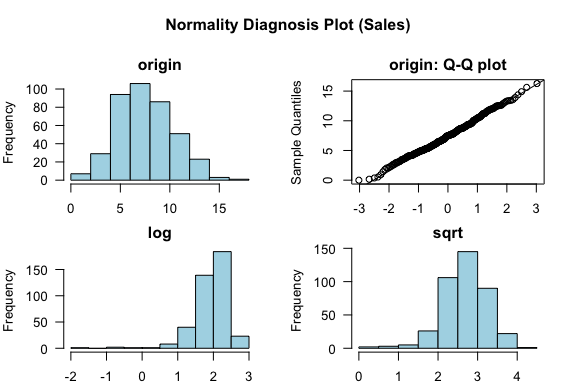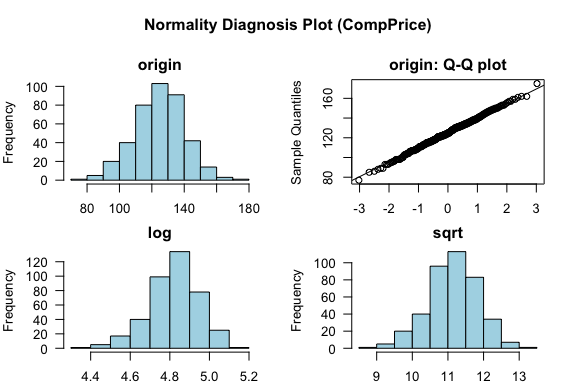The `plot_normality()` function also supports the `group_by()` function syntax in the `dplyr` package.

``````carseats %>%
filter(ShelveLoc == "Good") %>%
group_by(US) %>%
plot_normality(Income)``````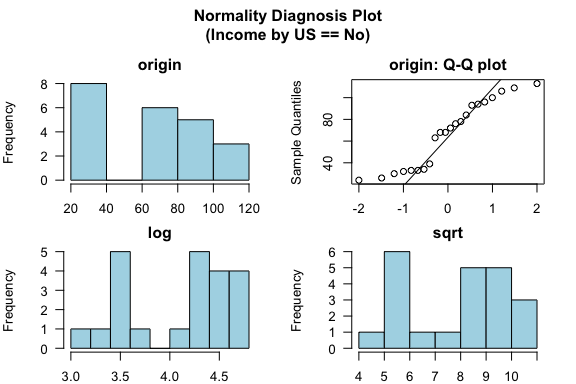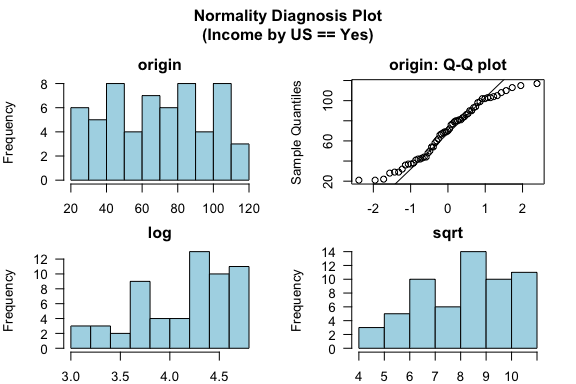## Bivariate data EDA

### Calculation of `correlation coefficient` using `correlate()`

`Correlate()` finds the correlation coefficient of all combinations of `carseats` numerical variables as follows:

``````correlate(carseats)
# A tibble: 56 x 3
var1        var2  coef_corr
<fct>       <fct>     <dbl>
1 CompPrice   Sales    0.0641
2 Income      Sales    0.151
4 Population  Sales    0.0505
# … with 52 more rows``````

The following example performs a normality test only on combinations that include several selected variables.

``````# Select columns by name
correlate(carseats, Sales, CompPrice, Income)
# A tibble: 21 x 3
var1      var2      coef_corr
<fct>     <fct>         <dbl>
1 CompPrice Sales        0.0641
2 Income    Sales        0.151
3 Sales     CompPrice    0.0641
4 Income    CompPrice   -0.0761
# … with 17 more rows

# Select all columns between year and day (inclusive)
correlate(carseats, Sales:Income)
# A tibble: 21 x 3
var1      var2      coef_corr
<fct>     <fct>         <dbl>
1 CompPrice Sales        0.0641
2 Income    Sales        0.151
3 Sales     CompPrice    0.0641
4 Income    CompPrice   -0.0761
# … with 17 more rows

# Select all columns except those from year to day (inclusive)
correlate(carseats, -(Sales:Income))
# A tibble: 35 x 3
var1        var2  coef_corr
<fct>       <fct>     <dbl>
2 Population  Sales    0.0505
3 Price       Sales   -0.445
4 Age         Sales   -0.232
# … with 31 more rows``````

`correlate()` produces `two pairs of variable` combinations. So you can use the following `filter()` function to get the correlation coefficient for `a pair of variable` combinations:

``````carseats %>%
correlate(Sales:Income) %>%
filter(as.integer(var1) > as.integer(var2))
# A tibble: 3 x 3
var1      var2      coef_corr
<fct>     <fct>         <dbl>
1 CompPrice Sales        0.0641
2 Income    Sales        0.151
3 Income    CompPrice   -0.0761``````

The `correlate()` function also supports the `group_by()` function syntax in the `dplyr` package.

``````carseats %>%
filter(ShelveLoc == "Good") %>%
group_by(Urban, US) %>%
correlate(Sales) %>%
filter(abs(coef_corr) > 0.5)
Warning: Factor `Urban` contains implicit NA, consider using
`forcats::fct_explicit_na`
# A tibble: 10 x 5
Urban US    var1  var2       coef_corr
<fct> <fct> <fct> <fct>          <dbl>
1 No    No    Sales Population    -0.530
2 No    No    Sales Price         -0.838
3 No    Yes   Sales Price         -0.630
4 Yes   No    Sales Price         -0.833
# … with 6 more rows``````

### Visualization of the correlation matrix using `plot_correlate()`

`plot_correlate()` visualizes the correlation matrix.

``plot_correlate(carseats)``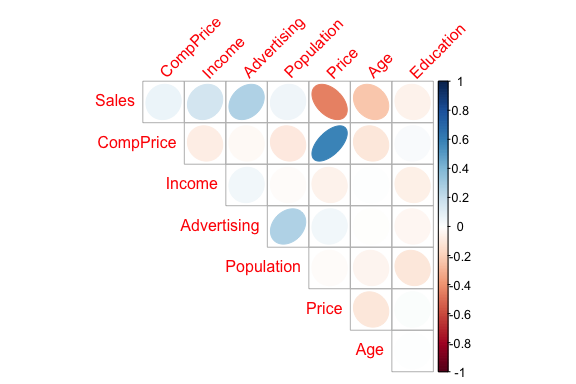`plot_correlate()` can also specify multiple variables, like the `correlate()` function. The following is a visualization of the correlation matrix including several selected variables.

``````# Select columns by name
plot_correlate(carseats, Sales, Price)``````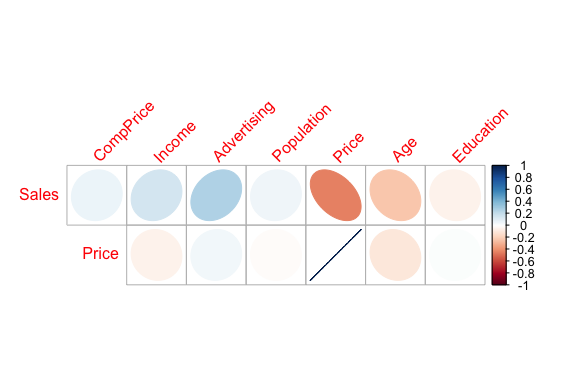The `plot_correlate()` function also supports the `group_by()` function syntax in the `dplyr` package.

``````carseats %>%
filter(ShelveLoc == "Good") %>%
group_by(Urban, US) %>%
plot_correlate(Sales)``````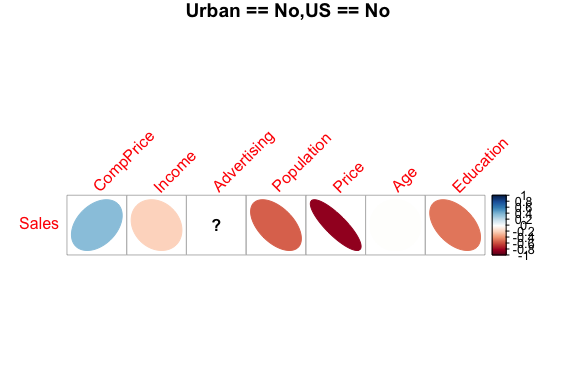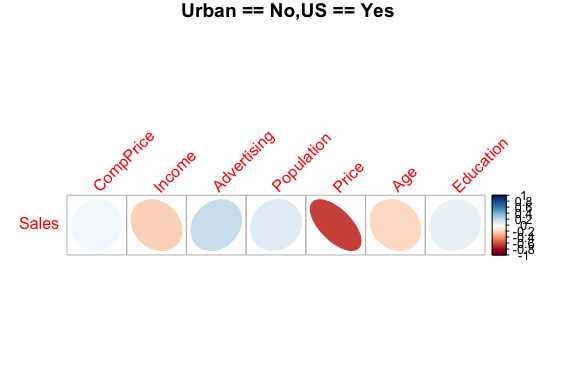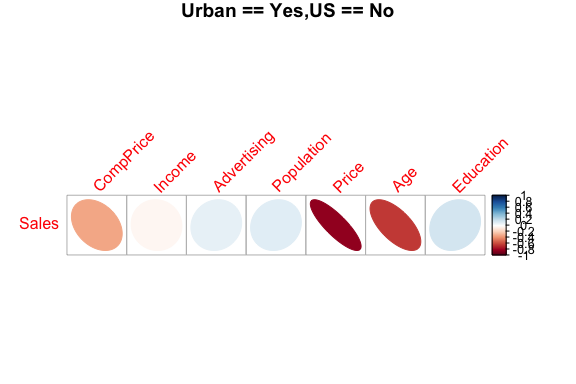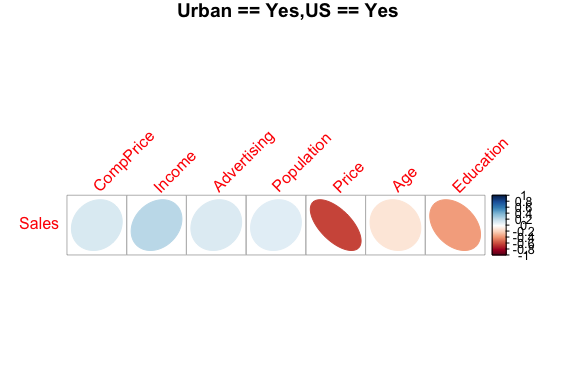## EDA based on target variable

### Definition of target variable

To perform EDA based on `target variable`, you need to create a`target_by class` object. `target_by()` creates a target_by class with an object inheriting data.frame or data.frame. `target_by()` is similar to `group_by()` in `dplyr` which creates`grouped_df`. The difference is that you specify only one variable.

The following is an example of specifying US as target variable in carseats data.frame.:

``````categ <- target_by(carseats, US)
Warning: Unquoting language objects with `!!!` is deprecated as of rlang 0.4.0.

dplyr::select(data, !!!enquo(x))

# Good:
dplyr::select(data, !!enquo(x))    # Unquote single quosure
dplyr::select(data, !!!enquos(x))  # Splice list of quosures

This warning is displayed once per session.``````

### EDA when target variable is categorical variable

Let’s do the EDA when the target variable is categorical. When the categorical variable US is the target variable, the relationship between the target variable and the predictor is examined.

#### Cases where predictors are numeric variable

`relate()` shows the relationship between the target variable and the predictor. The following example shows the relationship between Sales and the target variable US. The predictor Sales is a numeric variable. In this case, the descriptive statistics are shown for each level of the target variable.

``````# If the variable of interest is a numarical variable
cat_num <- relate(categ, Sales)
cat_num
# A tibble: 3 x 27
variable US        n    na  mean    sd se_mean   IQR skewness kurtosis   p00
<chr>    <fct> <dbl> <dbl> <dbl> <dbl>   <dbl> <dbl>    <dbl>    <dbl> <dbl>
1 Sales    No      142     0  6.82  2.60   0.218  3.44   0.323    0.808   0
2 Sales    Yes     258     0  7.87  2.88   0.179  4.23   0.0760  -0.326   0.37
3 Sales    total   400     0  7.50  2.82   0.141  3.93   0.186   -0.0809  0
# … with 16 more variables: p01 <dbl>, p05 <dbl>, p10 <dbl>, p20 <dbl>,
#   p25 <dbl>, p30 <dbl>, p40 <dbl>, p50 <dbl>, p60 <dbl>, p70 <dbl>,
#   p75 <dbl>, p80 <dbl>, p90 <dbl>, p95 <dbl>, p99 <dbl>, p100 <dbl>
summary(cat_num)
variable             US          n               na         mean
Length:3           No   :1   Min.   :142.0   Min.   :0   Min.   :6.823
Class :character   Yes  :1   1st Qu.:200.0   1st Qu.:0   1st Qu.:7.160
Mode  :character   total:1   Median :258.0   Median :0   Median :7.496
Mean   :266.7   Mean   :0   Mean   :7.395
3rd Qu.:329.0   3rd Qu.:0   3rd Qu.:7.682
Max.   :400.0   Max.   :0   Max.   :7.867
sd           se_mean            IQR           skewness
Min.   :2.603   Min.   :0.1412   Min.   :3.442   Min.   :0.07603
1st Qu.:2.713   1st Qu.:0.1602   1st Qu.:3.686   1st Qu.:0.13080
Median :2.824   Median :0.1791   Median :3.930   Median :0.18556
Mean   :2.768   Mean   :0.1796   Mean   :3.866   Mean   :0.19489
3rd Qu.:2.851   3rd Qu.:0.1988   3rd Qu.:4.077   3rd Qu.:0.25432
Max.   :2.877   Max.   :0.2184   Max.   :4.225   Max.   :0.32308
kurtosis             p00              p01              p05
Min.   :-0.32638   Min.   :0.0000   Min.   :0.4675   Min.   :3.147
1st Qu.:-0.20363   1st Qu.:0.0000   1st Qu.:0.6868   1st Qu.:3.148
Median :-0.08088   Median :0.0000   Median :0.9062   Median :3.149
Mean   : 0.13350   Mean   :0.1233   Mean   :1.0072   Mean   :3.183
3rd Qu.: 0.36344   3rd Qu.:0.1850   3rd Qu.:1.2771   3rd Qu.:3.200
Max.   : 0.80776   Max.   :0.3700   Max.   :1.6480   Max.   :3.252
p10             p20             p25             p30
Min.   :3.917   Min.   :4.754   Min.   :5.080   Min.   :5.306
1st Qu.:4.018   1st Qu.:4.910   1st Qu.:5.235   1st Qu.:5.587
Median :4.119   Median :5.066   Median :5.390   Median :5.867
Mean   :4.073   Mean   :5.051   Mean   :5.411   Mean   :5.775
3rd Qu.:4.152   3rd Qu.:5.199   3rd Qu.:5.576   3rd Qu.:6.010
Max.   :4.184   Max.   :5.332   Max.   :5.763   Max.   :6.153
p40             p50             p60             p70
Min.   :5.994   Min.   :6.660   Min.   :7.496   Min.   :7.957
1st Qu.:6.301   1st Qu.:7.075   1st Qu.:7.787   1st Qu.:8.386
Median :6.608   Median :7.490   Median :8.078   Median :8.815
Mean   :6.506   Mean   :7.313   Mean   :8.076   Mean   :8.740
3rd Qu.:6.762   3rd Qu.:7.640   3rd Qu.:8.366   3rd Qu.:9.132
Max.   :6.916   Max.   :7.790   Max.   :8.654   Max.   :9.449
p75             p80              p90              p95
Min.   :8.523   Min.   : 8.772   Min.   : 9.349   Min.   :11.28
1st Qu.:8.921   1st Qu.: 9.265   1st Qu.:10.325   1st Qu.:11.86
Median :9.320   Median : 9.758   Median :11.300   Median :12.44
Mean   :9.277   Mean   : 9.665   Mean   :10.795   Mean   :12.08
3rd Qu.:9.654   3rd Qu.:10.111   3rd Qu.:11.518   3rd Qu.:12.49
Max.   :9.988   Max.   :10.464   Max.   :11.736   Max.   :12.54
p99             p100
Min.   :13.64   Min.   :14.90
1st Qu.:13.78   1st Qu.:15.59
Median :13.91   Median :16.27
Mean   :13.86   Mean   :15.81
3rd Qu.:13.97   3rd Qu.:16.27
Max.   :14.03   Max.   :16.27  ``````

The `relate class` object created with`relate()` visualizes the relationship between the target variable and the predictor with `plot()`. The relationship between US and Sales is represented by a density plot.

``plot(cat_num)``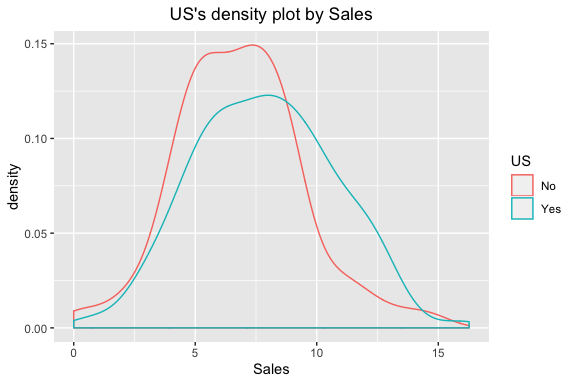#### Cases where predictors are categorical variable

The following example shows the relationship between `ShelveLoc` and the target variable `US`. The predictor, ShelveLoc, is a categorical variable. In this case, we show the `contigency table` of two variables. The `summary()` function also performs an `independence test` on the contigency table.

``````# If the variable of interest is a categorical variable
cat_cat <- relate(categ, ShelveLoc)
cat_cat
ShelveLoc
No   34   24     84
Yes  62   61    135
summary(cat_cat)
Call: xtabs(formula = formula_str, data = data, addNA = TRUE)
Number of cases in table: 400
Number of factors: 2
Test for independence of all factors:
Chisq = 2.7397, df = 2, p-value = 0.2541``````

`plot()` visualizes the relationship between the target variable and the predictor. The relationship between `US` and `ShelveLoc` is represented by a `mosaics plot`.

``plot(cat_cat)``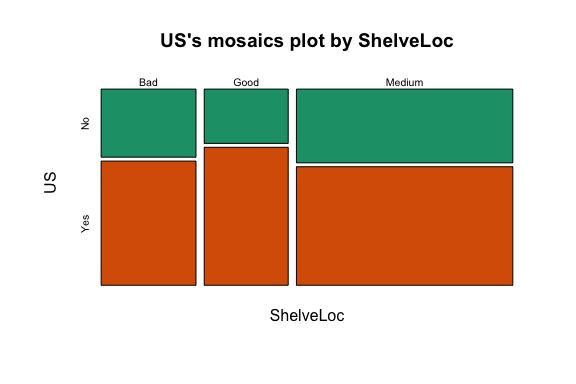### EDA when target variable is numerical variable

Let’s do the EDA when the target variable is numeric. When the numeric variable Sales is the target variable, the relationship between the target variable and the predictor is examined.

``````# If the variable of interest is a numarical variable
num <- target_by(carseats, Sales)``````

#### Cases where predictors are numeric variable

The following example shows the relationship between `Price` and the target variable `Sales`. Price, a predictor, is a numeric variable. In this case, we show the result of `simple regression model` of `target ~ predictor` relation. The `summary()` function represents the details of the model.

``````# If the variable of interest is a numarical variable
num_num <- relate(num, Price)
num_num

Call:
lm(formula = formula_str, data = data)

Coefficients:
(Intercept)        Price
13.64192     -0.05307
summary(num_num)

Call:
lm(formula = formula_str, data = data)

Residuals:
Min      1Q  Median      3Q     Max
-6.5224 -1.8442 -0.1459  1.6503  7.5108

Coefficients:
Estimate Std. Error t value Pr(>|t|)
(Intercept) 13.641915   0.632812  21.558   <2e-16 ***
Price       -0.053073   0.005354  -9.912   <2e-16 ***
---
Signif. codes:  0 '***' 0.001 '**' 0.01 '*' 0.05 '.' 0.1 ' ' 1

Residual standard error: 2.532 on 398 degrees of freedom
Multiple R-squared:  0.198, Adjusted R-squared:  0.196
F-statistic: 98.25 on 1 and 398 DF,  p-value: < 2.2e-16``````

`plot()` visualizes the relationship between the target variable and the predictor. The relationship between Sales and Price is repersented as a scatter plot. The plot on the left represents the scatter plot of Sales and Price and the confidence interval of the regression line and the regression line. The plot on the right represents the relationship between the original data and the predicted value of the linear model as a scatter plot. If there is a linear relationship between the two variables, the observations will converge on the red diagonal in the scatter plot.

``plot(num_num)``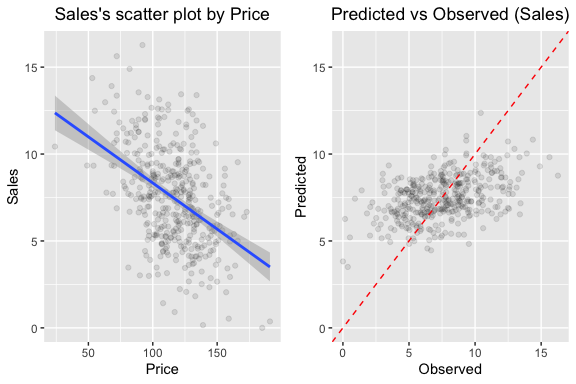The scatter plot of the data with a large number of observations is output as overlapping points. This makes it difficult to judge the relationship between the two variables. It also takes a long time to perform the visualization. In this case, the above problem can be solved by hexabin plot.

In `plot()`, the hex_thres argument provides a basis for drawing hexabin plots. For data with more than this number of observations, draw a hexabin plot.

Next, draw a hexabin plot with `plot()` not a scatter plot, specifying 350 for the hex_thres argument. This is because the number of observations is 400.

``plot(num_num, hex_thres = 350)``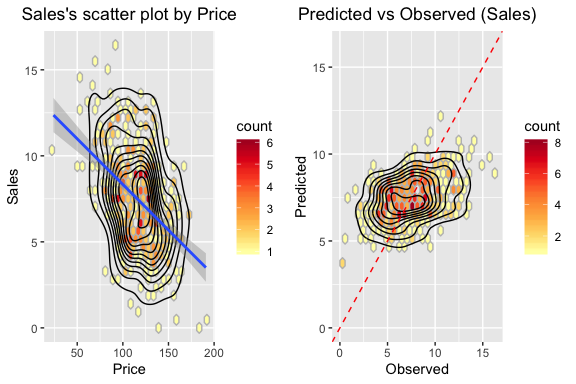#### Cases where predictors are categorical variable

The following example shows the relationship between `ShelveLoc` and the target variable `Sales`. The predictor, ShelveLoc, is a categorical variable. It shows the result of performing `one-way ANOVA` of `target ~ predictor` relation. The results are represented in terms of an analysis of variance. The `summary()` function also shows the `regression coefficients` for each level of the predictor. In other words, it shows detailed information of `simple regression analysis` of `target ~ predictor` relation.

``````# If the variable of interest is a categorical variable
num_cat <- relate(num, ShelveLoc)
num_cat
Analysis of Variance Table

Response: Sales
Df Sum Sq Mean Sq F value    Pr(>F)
ShelveLoc   2 1009.5  504.77   92.23 < 2.2e-16 ***
Residuals 397 2172.7    5.47
---
Signif. codes:  0 '***' 0.001 '**' 0.01 '*' 0.05 '.' 0.1 ' ' 1
summary(num_cat)

Call:
lm(formula = formula(formula_str), data = data)

Residuals:
Min      1Q  Median      3Q     Max
-7.3066 -1.6282 -0.0416  1.5666  6.1471

Coefficients:
Estimate Std. Error t value Pr(>|t|)
(Intercept)       5.5229     0.2388  23.131  < 2e-16 ***
ShelveLocGood     4.6911     0.3484  13.464  < 2e-16 ***
ShelveLocMedium   1.7837     0.2864   6.229  1.2e-09 ***
---
Signif. codes:  0 '***' 0.001 '**' 0.01 '*' 0.05 '.' 0.1 ' ' 1

Residual standard error: 2.339 on 397 degrees of freedom
Multiple R-squared:  0.3172,    Adjusted R-squared:  0.3138
F-statistic: 92.23 on 2 and 397 DF,  p-value: < 2.2e-16``````

`plot()` visualizes the relationship between the target variable and the predictor. The relationship between `Sales` and `ShelveLoc` is represented by a `box plot`.

``plot(num_cat)``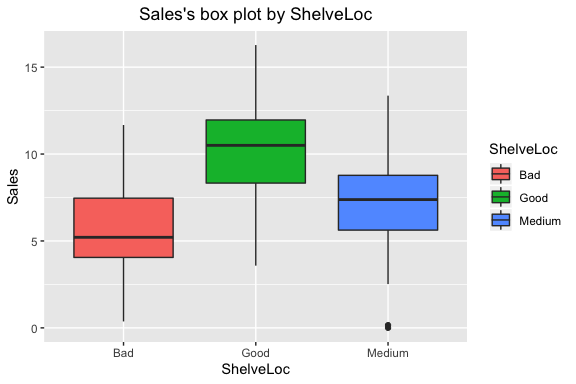## Creating an EDA report using `eda_report()`

`eda_report()` performs EDA on all variables of the data frame or object (`tbl_df`,`tbl`, etc.) that inherits the data frame.

`eda_report()` creates an EDA report in two forms:

• pdf file based on Latex
• html file

The contents of the report are as follows.:

• introduction
• Information of Dataset
• Information of Variables
• Numerical Variables
• Univariate Analysis
• Descriptive Statistics
• Normality Test of Numerical Variables
• Statistics and Visualization of (Sample) Data
• Relationship Between Variables
• Correlation Coefficient
• Correlation Coefficient by Variable Combination
• Correlation Plot of Numerical Variables
• Target based Analysis
• Gruoped Descriptive Statistics
• Gruoped Numerical Variables
• Gruoped Categorical Variables
• Gruoped Relationship Between Variables
• Grouped Correlation Coefficient
• Grouped Correlation Plot of Numerical Variables

The following will create an EDA report for `carseats`. The file format is pdf, and the file name is `EDA_Report.pdf`.

``````carseats %>%
eda_report(target = Sales)``````

The following generates an HTML-formatted report named `EDA.html`.

``````carseats %>%
eda_report(target = Sales, output_format = "html", output_file = "EDA.html")``````

The EDA report is an automated report to assist in the EDA process. Design the data analysis scenario with reference to the report results.

### EDA report contents

#### Contents of pdf file

• The cover of the report is shown in the following figure.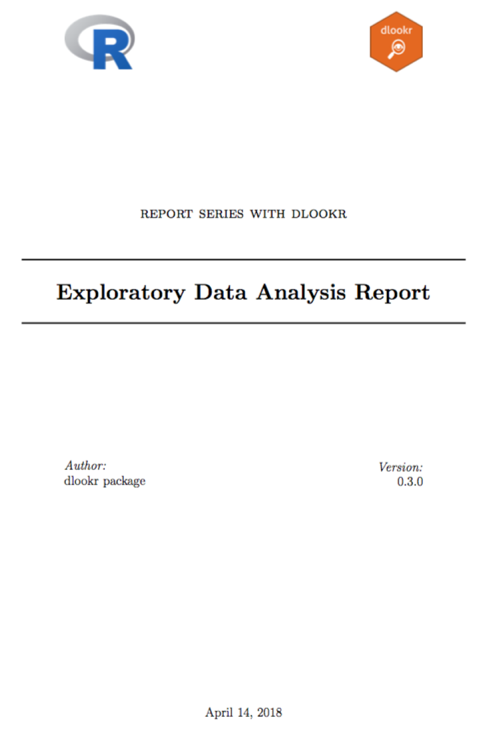EDA report cover

• The report’s argenda is shown in the following figure.